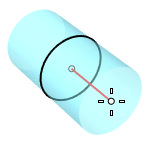# MeshCylinderMesh

Polygon Mesh Primitives >

Cylinder

The MeshCylinder command draws a polygon mesh cylinder.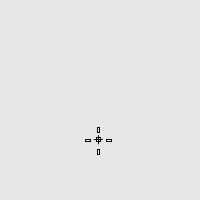#### Steps

1. Draw the base circle.

2. Pick a location or type a number to set the height.

The DirectionConstraint options define the positive height differently.

Command-line options

### DirectionConstraint

Direction constraints restrict the direction of the cylinder.

##### None

Pick or type a number to set the height.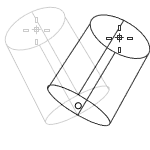##### Vertical

Creates a cylinder perpendicular to the construction plane.• The CPlane +Z direction defines the positive direction when you type a number to set the height.
##### AroundCurve

Draws the base circle perpendicular to the picked point on a curve. The center line of the cylinder will be tangent to the curve.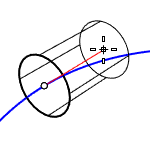• The curve direction defines the positive direction when you type a number to set the height.

### Solid

Caps the top and bottom openings or leave them open.Draws the base circle by picking the center point and a radius point.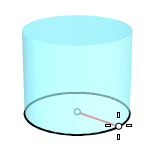### 2Point

Draws the base circle from two opposite points.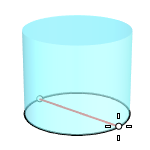### 3Point

Draws the base circle through three points.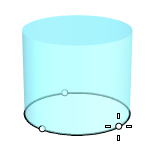### Tangent

Draws the base circle tangent to one, two, or three curves.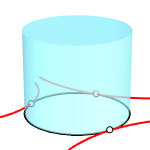### FitPoints

Draws the base circle by fitting to selected points, control points, or mesh vertices.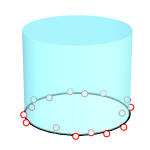### AroundFaces

The number of faces around the circumference.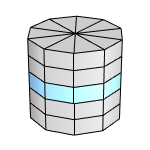### VerticalFaces

The number of faces from the base to the apex.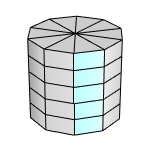### BothSides

Creates a cylinder symmetrical across the base circle. The height of the cylinder is twice as long as you specify.